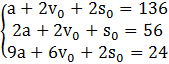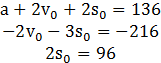### Sample Problem

A ball is thrown vertically in the air. Find the ball’s position equation s=0.5at2+v0t+s0

Given that at t=1 second, s= 68 feet

At t=2 seconds, s=56 feet

At t=3 seconds, s=12 feet

S=t2+t+ (round all answers to the nearest whole number)

#### Solution

Plug in all the given values to create a system of equations:

s=0.5at2+v0t+s0

68=0.5a(1)2+v0(1)+s0=0.5a+v0+s0 à 136=a+2v0+2s0

56=0.5a(2)2+v0(2)+s0=2a+2v0+s0

12=0.5a(3)2+v0(3)+s0=4.5a+3v0+s0 à 24=9a+6v0+2s0Use Gaussian elimination to solve for the variables:E2=E2-2E1

E3=E3-9E1E3=E3-6E2E2=E2/-2

E1=E1/2By back substituting s0=48 into the second equation, we can find v0:

v0+3/2s0=108

v0=108-3/2s0=108-3/2(48)=36

By back substituting s0=48 and v0=36 into the first equation, we can find a:

a+2v0+2s0=136

a=136-2v0-2s0=136-2(36)-2(48)=-32

Therefore, plugging in the values of a, s0, and v0 in the position equation:

s=0.5at2+v0t+s0

S=0.5(-32)t2+36t+48=-16t2+36+48